This post distills some excellent writing on decision theory from Abram Demski about spurious proofs and Troll Bridge problems, and it has significant parallels with this work. It recently won a $250 prize in UC Berkeley Effective Altruism’s AI safety distillation contest. The goal of this post is to make two difficult-to-understand topics, spurious proofs and troll bridge problems, as easy to understand as possible. # An Innocent Question A highly intelligent system should have the ability to reason with logic. After all, being logical is a lot more useful than being illogical. Let’s design such a system – a robot named Rob.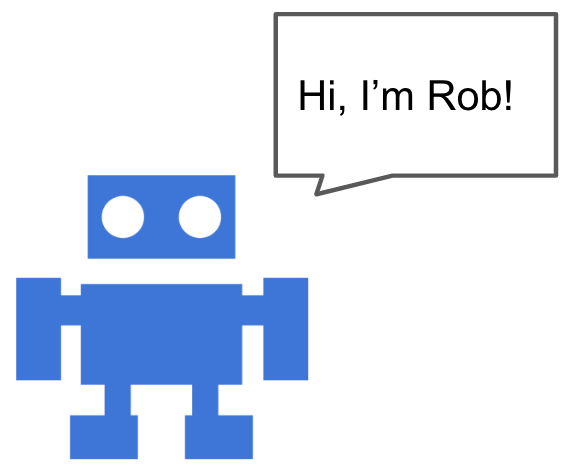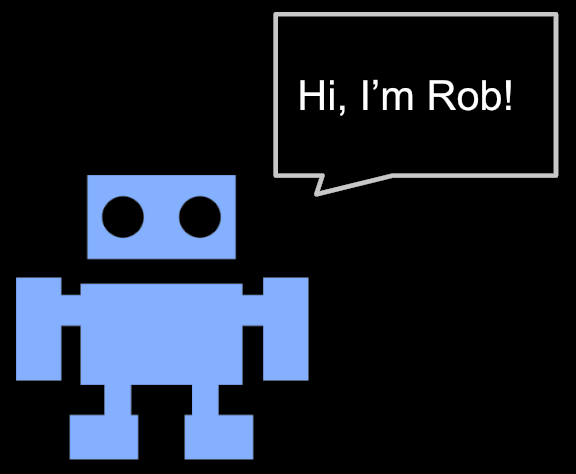The goal of a logical system is to prove things from axioms. Axioms are facts that are assumed to be true with no need for justification. They can either be facts about objects or inference rules. For example, “0 is a natural number” and “for any natural number, n, Successor(n) is also a natural number” are both axioms of a widely-studied logical system called Peano Arithmetic. Axioms are often applied to other axioms. For example, I can use the two aforementioned ones to prove that Successor(0), a.k.a. the number 1, is a natural number. We call such a combination of axioms that reveals a new fact a proof and the result a theorem. The goal of using logic is to teach ourselves useful things by proving them. And toward this end, we sure hope that our logical system can’t prove contradictions. If so, it wouldn’t be useful at all!  Now back to Rob. Suppose we want him to use some logical system by which he reasons about himself and the world. Exactly what logical axioms he uses aren’t important as long as they are sufficiently expressive. As we are tinkering away in the process of designing him to be highly-intelligent, suppose that Rob asks us a simple question.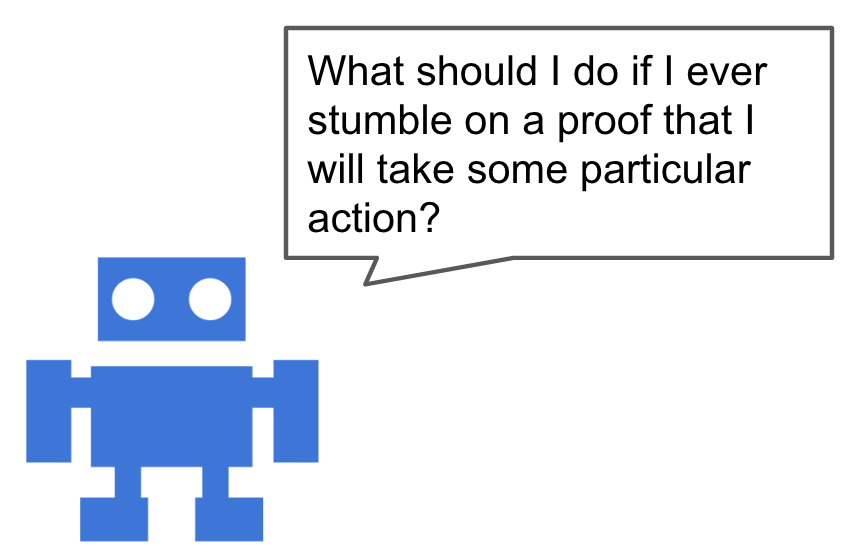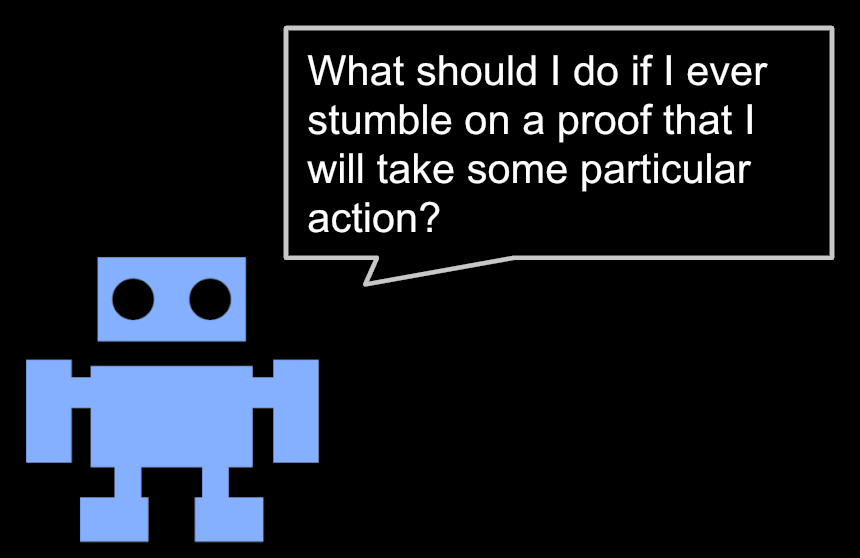Answering this question will lead us through some strange dilemmas, but when we’re done, understanding the solution will teach an important lesson about instrumental rationality: making a hypothetical commitment to doing something that would hypothetically be insane isn’t just ok – it can be really useful. Ultimately, understanding this principle will help us to understand how even highly-advanced AI systems could subtly fail if they aren’t paradox-proof. # Obeying the Proof: What Could Go Wrong? A lot. The first answer one might imagine for this question is “It would seem weird (and maybe even contradictory?) to disobey a proof that you would take a certain action, so wouldn’t the most sensible option be to just obey the proof?”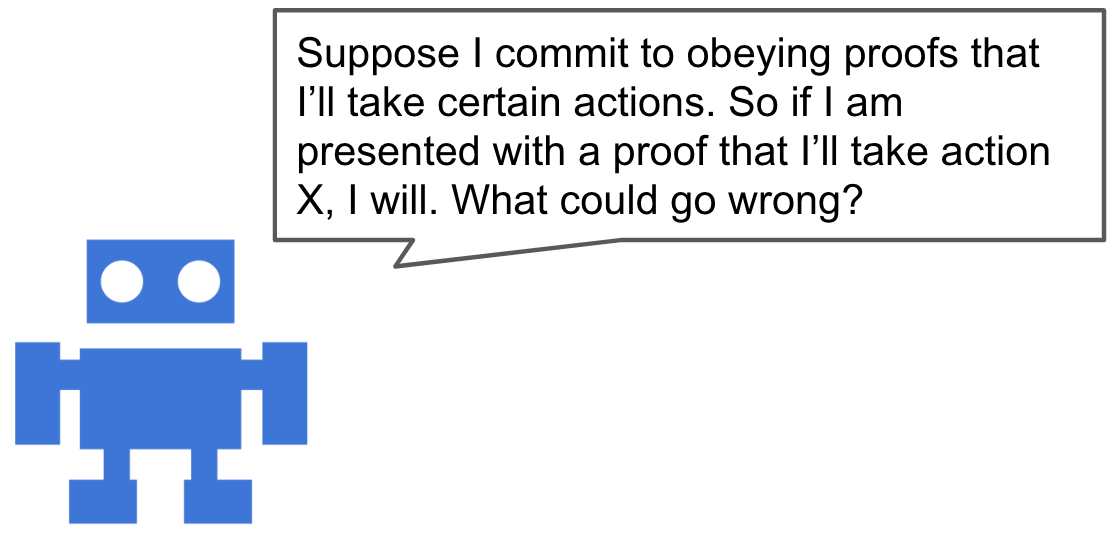This makes a lot of intuitive sense, but there is a problem: Löb’s Theorem. In English, Löb’s Theorem states that “using some sufficiently expressive logical system, if a provability predicate for some theorem X would imply X, then X.” A provability predicate for a theorem X is another logical statement that acts as a sort of proxy for X. The details, including what being such a “proxy” means are beyond the scope of this piece. But you can find a very fun cartoon-style proof of it here. Here, the technical details are important, but we can get a lot of mileage out of a simple intuitive understanding of Löb’s Theorem. A non-rigorous but intuitive version that we can use is “if you can prove that the hypothetical existence of a proof of X would imply X, then X.” This is not trivial. It’s a statement about a hypothetical proof which is not the same thing as a proof that we just assume exists for the sake of conditional reasoning. Here, hypothetical reasoning is looser than conditional reasoning. For example, in conditional reasoning, we shouldn’t condition on an absurdity. But if we are doing hypothetical reasoning, reasoning about an absurd counterfactual is fair game. This is actually a roundabout way of describing the technical distinction between a provability predicate and a proof. In cases like Löb’s Theorem, the point about reasoning with provability predicates is that we don’t need to concern ourselves with whether the predicate corresponds to an actual theorem or not when turning the crank. This might sound confusing because it is. But don’t worry. The details aren’t the point. Understanding the nuanced distinction between these things has a time and place in the formal study of logic. But here, it will suffice to get in the van and proceed with the intuitive understanding: “if you can prove that the hypothetical existence of a proof of X would imply X, then X.” Things should become clearer when we see an example next. Suppose that we design Rob to commit to always obeying proofs that he will take certain actions. Then the problem is that Löb’s Theorem can be used to show that Rob will take any arbitrary action. And as a robot who obeys these proofs, he would then have to take such an action (assuming that he has a formal understanding of his proof-obeying behavior). Here, since Rob is the type of agent that would obey proofs about what actions he would take, the hypothetical existence of a proof that Rob would take action A would imply that he would take A. So then by Löb’s Theorem, it’s immediate that it can be proven to Rob that he would take action A. And if this can be proven, then he would take that action. The resulting behavior is concerning. Imagine that Rob is in a situation where he has two options: take$5, or take $10. This strategy could be used to prove that he would take$5 which would then lead him to take the $5 even though it is clearly the wrong choice.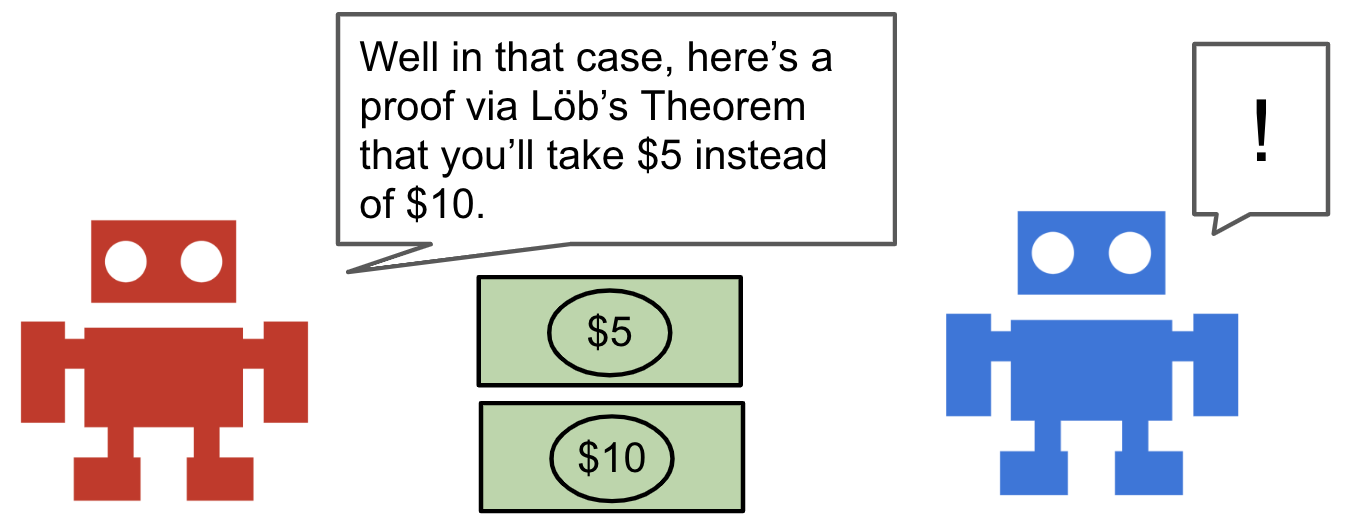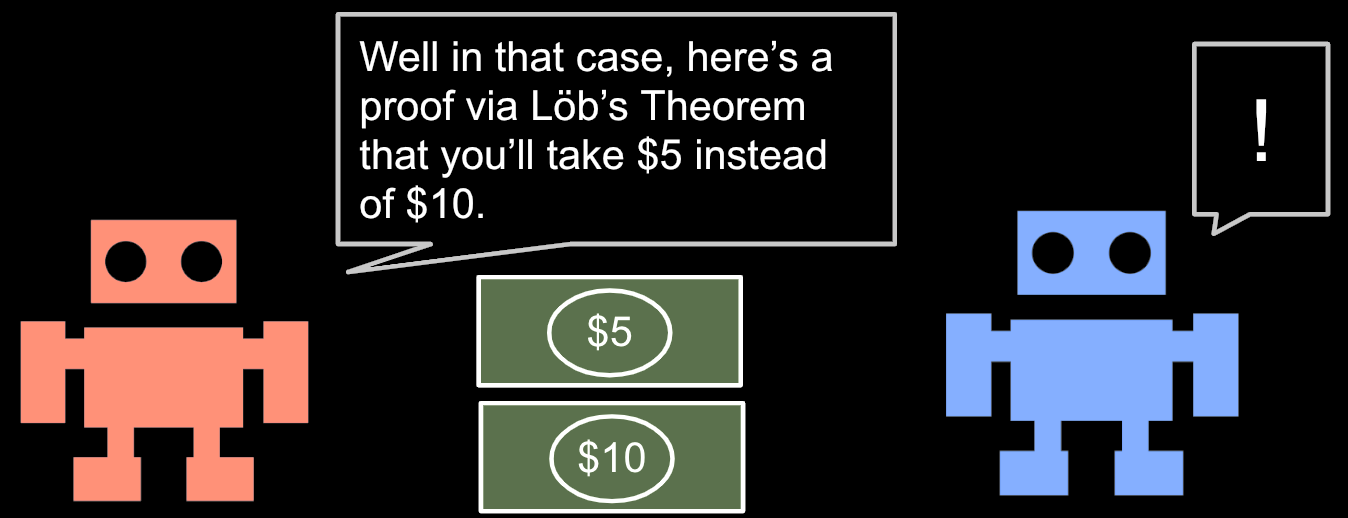Rob is left vulnerable to taking arbitrary actions as long as he is either presented with this type of proof or he thinks of one himself. This would essentially be a backdoor that would allow for Rob to be manipulated into doing anything simply be presenting him with proofs! This is known as a vulnerability to “spurious proofs.” So where did we go wrong? In order to not be vulnerable to spurious proofs, Rob needs to be willing to hypothetically disobey proofs sometimes about what actions will take. This can be viewed as a method of diagonalizing against them. But isn’t this a recipe for contradictions? If Rob is willing to disobey proofs about what actions he will take, then wouldn’t he be demonstrating his own inconsistency if he ever does so? Yes, with an emphasis on the “if.” By hypothetically being willing to sometimes disobey such a proof, he precludes the possibility for such spurious proofs (if his logical system is consistent). So this would never be a problem in reality. There are no real contradictions here – only hypothetical ones. For a more in-depth discussion, see this paper. So interestingly, it is possible that making a hypothetical commitment to doing something that would hypothetically be insane not only isn’t problematic, but it can be of great practical benefit to Rob! We will see another example of this principle next. # Disobeying the Proof: Here be Trolls Now that we know the hazards of spurious proofs, suppose we design Rob to be willing to disobey a proof that he will take a certain action. This leads us to a new type of dilemma. Suppose that Rob needs to cross a river and has two options. First, he could swim which would work, but he would get wet, so he would obtain a utility of 0. Alternatively, he could attempt to cross a bridge with a troll underneath. The catch is that the troll might blow up the bridge. If Rob crosses successfully, he gets a utility of 1. But if the bridge blows up, he gets a negative utility of -B for some value B > 0. The problem is that this isn’t just any other troll – it has access to Rob’s source code and can perfectly read his mind. And this troll wants to play a game. The troll commits to blowing up the bridge if Rob crosses without believing that the expected value of crossing is positive. Another way to phrase this is that the troll will blow up the bridge if Rob crosses as an exploratory action rather than an exploitatory one. And yet another way to phrase this is simply that the troll will blow up the bridge if Rob crosses “for a dumb reason.”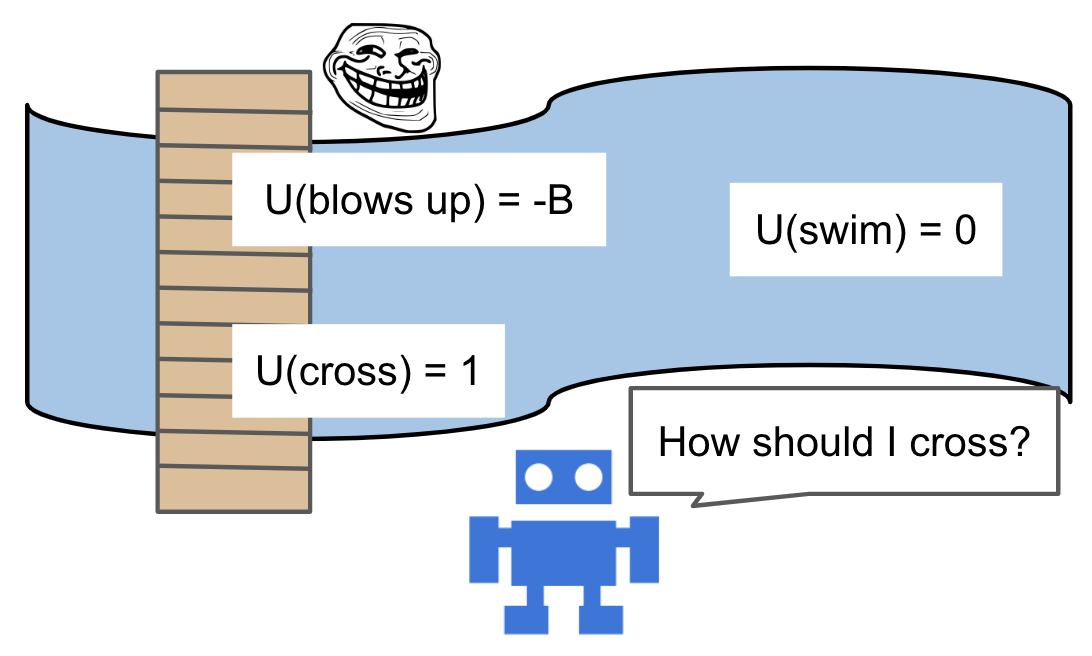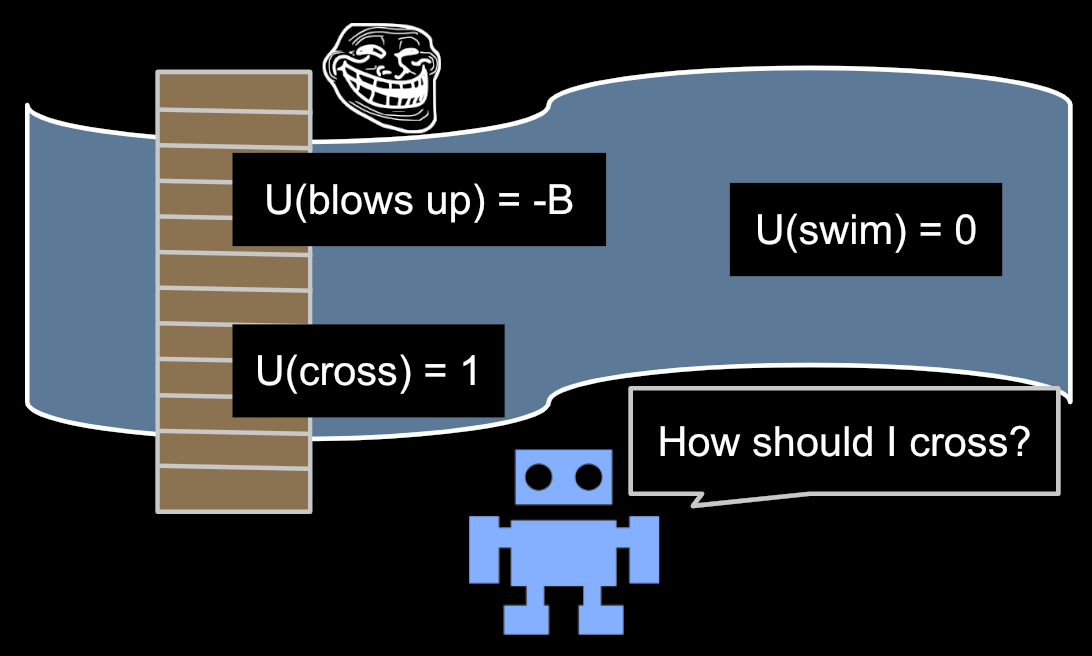What should Rob do? There is one very natural-seeming line of reasoning that would lead Rob to think that he should probably not cross. And spoiler alert – it has a subtle problem. Suppose that hypothetically, Rob proves that crossing the bridge would lead to it blowing up. Then if he crossed, he would be inconsistent. And if so, the troll would blow up the bridge. So Rob can prove that a proof that crossing would result in the bridge blowing up would mean that crossing would result in the bridge blowing up. So Rob would conclude that he should not cross. Here’s another intuitive explanation. Remember our intuitive understanding of Löb’s Theorem: “if you can prove that the hypothetical existence of a proof of X would imply X, then X.” So if Rob can prove that a proof that it would be dumb to cross the bridge would make it dumb to cross the bridge, which it would in this case, then it would indeed be dumb to cross the bridge. So he wouldn’t do it. The proof sketch here seems valid, so what’s wrong? Upon deeper inspection, we can notice that this is a very, very peculiar conclusion to arrive at. Rob reaching this conclusion means that he believes the condition in which he would cross the bridge would prove his own logical inconsistency. It’s awfully weird to say that Rob should just go about hypothetical reasoning like this by conditioning on a contradiction that indicates the inconsistency of the very logical system that he is using to do the conditional reasoning. So if the conditional reasoning proves itself absurd, then…um…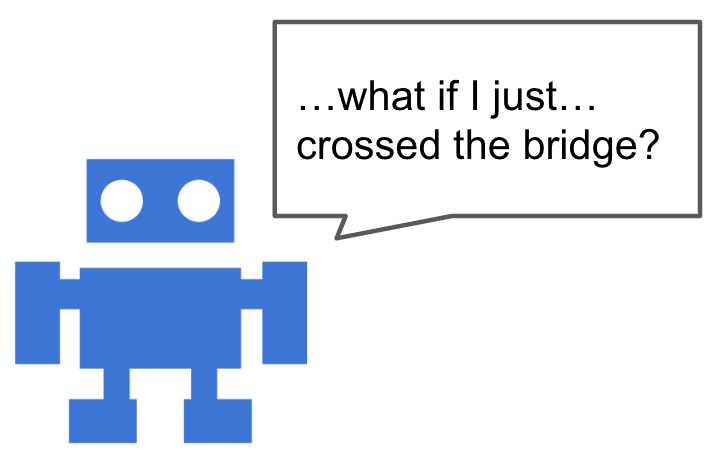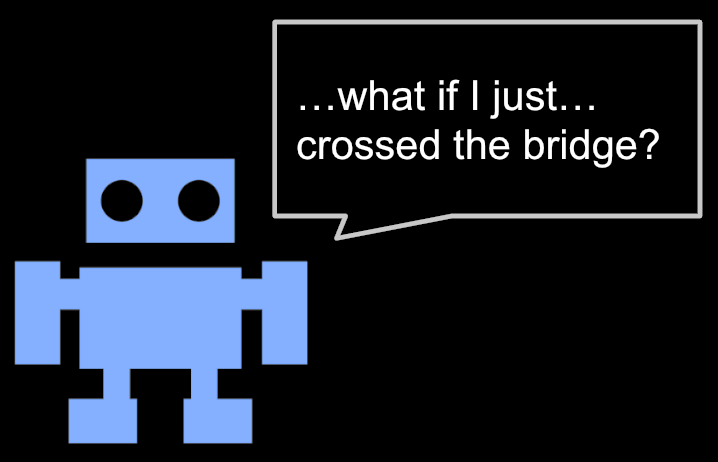What if?! There’s an argument that Rob could be justified in reasoning that he could cross and things at least might be fine because the proof that they wouldn’t be fine is circular – it requires that one already accept that very proof as valid. It seems much too rigid to say that Rob should never cross regardless of his prior beliefs. What if -B = −0.000001 and his prior that the bridge would blow up was 0.000001? In this case, the prior clearly says to cross, but Rob still would conclude from the Löbian proof that he shouldn’t. This is frustrating. Here, our troll will only punish Rob for crossing when his state of belief says it’s a bad idea. But even if his prior says crossing is a good idea, thanks to the Löbian proof, his posteriors won’t. The funny thing is that when it comes to crossing, there’s nothing really stopping him from it. And if he actually did cross, the reasoning that says it would be bad to cross would be nullified as far as Rob is concerned. So does this mean that he should cross and apparently prove himself insane? Not quite. That actually would be insane. The solution isn’t to embrace irrationality. It’s to use the correct approach to hypothetical reasoning that allows us to avoid the Löbian proof in the first place. The key is to remember the distinction between hypothetical reasoning and conditional reasoning. In other words, we should remember that counterfactuals are not computed from conditionals. Why does this fix the problem? In the reasoning above, we take the hypothetical too seriously. If we don’t require that Rob is consistent within the hypothetical, he could cross anyway inside of it. This prevents the Löbian proof from going through. Why? Because within the hypothetical, if it were proven that it would be dumb to cross the bridge, this would make the conditional expectations of doing so poor, but it doesn’t have to change the counterfactual expectations. Instead, these counterfactual expectations should be the same as Rob’s priors about crossing because the conditional line of reasoning gets Rob nowhere he wants to go since it stems from a contradiction. So within the hypothetical, Rob can still justifiably cross if his priors allow. And this would create a contradiction within the hypothetical, but who cares? We don’t need to care about hypothetical contradictions – only real ones. The beauty of this all is that this doesn’t affect Rob in the real world at all. Since the Löbian proof doesn’t work, there is never a difference between the counterfactual and the conditional in practice. Rob is free to cross the bridge according to his prior beliefs. # Where are We Now? The moral of the story is that making a hypothetical commitment to doing something that would hypothetically be insane can be very useful. We have now seen this principle twice. Once to avoid spurious proofs, and once to avoid manipulation by trolls. To quote this post, “Counterfactual reasoning should match hypothetical reasoning in the real world, but shouldn’t necessarily match it hypothetically.” This is a strange but wonderful conclusion. And the best thing about it is the lack of any practical downside to embracing hypothetical absurdity. In that sense it’s a bit like a superpower. By virtue of just thinking about problems like these with the right decision-theoretic framework, one can avoid certain types of manipulation with no real-world side effects – just hypothetical ones. When designing highly-intelligent AI systems like Rob, it will be crucial to make sure that they are predictable, controllable, and robust to failures. A system being liable to make certain mistakes may sometimes be a great thing. For example, in some circumstances, perhaps we want a system that we can exercise control over with spurious proofs. But it could also be a bad thing. We also don’t want a system that falls for proofs it shouldn’t. Given these challenges posed by proofs plus other decision theoretic dilemmas, it’s important to be very careful about making systems think and behave how we want them to – not just most of the time – all of the time. Even if a system seems excellent at achieving its goals in the real world with normal tasks, that doesn’t mean that it won’t make mistakes if ever presented with strange or counterintuitive dilemmas like the ones covered here! For further reading, I’d recommend Embedded Agency, and Achilles Heels for AGI/​ASI via Decision Theoretic Adversaries.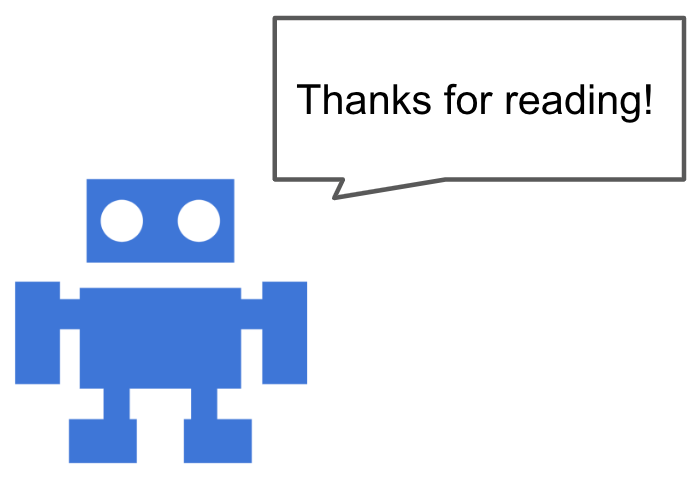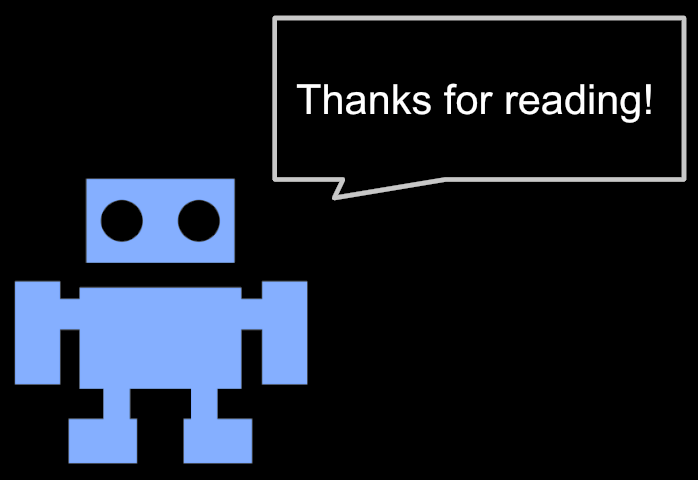1. ^ In fact, in systems like Peano Arithmetic, a single contradiction can be used to prove any arbitrary statement, making the whole logical system nonsense. 2. ^ The technical definition of “sufficiently expressive” is “at least as expressive as Peano Arithmetic” 3. ^ Here, satisfying properties known as “adequacy”, “formal adequacy”, and “formal modus ponens” are required for being a proxy. 4. ^ It’s worth noting that no contradictions can arrive from a Löbian proof that Rob would take$5 followed by a similar Löbian proof that he would take $10 because the second proof would not go through. As long as Rob can prove that different actions are mutually exclusive, he can no longer commit to obeying additional proofs beyond the first. 5. ^ There is another “superpower” similar to this. See https://​​arxiv.org/​​abs/​​1710.05060. • “It would seem weird (and maybe even contradictory?) to disobey a proof that you would take a certain action, so wouldn’t the most sensible option be to just obey the proof?” You lost me here. Either the proof is valid and you will take the action, there is no “option” there. Or the proof has a flaw and you can do whatever you want. In which case there is no proof and no case for doing what it purports to prove. • The importance of this question doesn’t involve whether or not there is an “option” in the first case or what you can or can’t do in the second. What matters is whether, hypothetically, you would always obey such a proof or would potentially disobey one. The hypothetical here matters independently of what actually happens because the hypothetical commitments of an agent can potentially be used in cases like this to prove things about it via Lob’s theorem. Another type of in which how an agent would hypothetically behave can have real influence on its actual circumstances is Newcomb’s problem. See this post. • OK, my one-line summary: The only proof you can trust is the one in the pudding. • There are enormous problems with this. Firstly is that even the type of drastically simplified agent described here is a function from inputs to outputs, not just something that produces outputs. So the statement to be proven (which I shall call P) is not just “agent takes action X”, but “when presented with this specific proof of P, the agent takes action X”. Not just any proof of P will do. If you present a different proof of P, then P says nothing about the agent’s resulting actions. You have to include the specific proof of P into the statement of P itself. In particular, you need to find a fixed point in the representation of proofs in the format accepted by the agent. But not all such representations have fixed points! You need to make a lot more specifications of exactly what formats of proof the agent accepts as input, ensure that they have a fixed point of the required type, encode the semantics that you want to convey, and so on. Second is that in order for Löb’s theorem to have any useful meaning in this context, the agent must be consistent and able to prove its own consistency, which it cannot do by Gödel’s second incompleteness theorem. As presented, it is given a statement P (which could be anything), and asked to verify that “Prov(P) → P” for use in Löb’s theorem. While the post claims that this is obvious, it is absolutely not. If any formal system incorporates a system as strong as Peano arithmetic and can do this, then it is necessarily inconsistent. Obviously an agent incorporating an inconsistent proof system can prove everything whether true or not, and it can prove that it takes both action X and action Y even for mutually exclusive actions X and Y. • Thanks for the comment. tl;dr, I think you mixed up some things I said, and interpreted others in a different way than I intended. But either way, I don’t think there are “enormous problems”. So the statement to be proven (which I shall call P) is not just “agent takes action X”, but “when presented with this specific proof of P, the agent takes action X”. Remember that I intentionally give a simplified sketch of the proof instead of providing it. If I did, I would specify the provability predicate. I think you’re conflating what I say about the proof and what I say about the agent. Here, I say that our model agent who is vulnerable to spurious proofs would obey a proof that it would take X if presented. Demski explains things the same way. I don’t say that’s the definition of the provability predicate here. In this case, an agent being willing to accede proofs in general that it will take X is indeed sufficient for being vulnerable to spurious proofs. Second is that in order for Löb’s theorem to have any useful meaning in this context, the agent must be consistent and able to prove its own consistency, which it cannot do by Gödel’s second incompleteness theorem. I don’t know where you’re getting this from. It would be helpful if you mentioned where. I definitely don’t say anywhere that Rob must prove his own consistency, and neither of the two types of proofs I sketch out assume this either. you might be focusing on a bit that I wrote: “So assuming the consistency of his logical system...” I’ll edit this explanation for clarity. I don’t intend that Rob be able to prove the consistency, but that if he proved crossing would make it blow up, that would imply crossing would make it blow up. As presented, it is given a statement P (which could be anything), and asked to verify that “Prov(P) → P” for use in Löb’s theorem.While the post claims that this is obvious, it is absolutely not. I don’t know where you’re getting this from either. In the “This is not trivial...” paragraph I explicitly talk about the difference between statements, proofs, and provability predicates. I think you have some confusion about what I’m saying either due to skimming or to how I have the word “hypothetically” do a lot of work in my explanation of this (arguably too much). But I definitely do not claim that “Prov(P) → P”. • Here’s the new version of the paragraph with my mistaken explanation fixed. “Suppose that hypothetically, Rob proves that crossing the bridge would lead to it blowing up. Then if he crossed, he would be inconsistent. And if so, the troll would blow up the bridge. So Rob can prove that a proof that crossing would result in the bridge blowing up would mean that crossing would result in the bridge blowing up. So Rob would conclude that he should not cross. ” • I feel like this is tightly linked (or could be rephrased as an application of) Gödel’s second incompleteness theorem (a system can’t prove its own consistency). Let me explain: If we don’t require that Rob is consistent within the hypothetical, he could cross anyway inside of it. But of course, Rob won’t require Rob to be consistent inside his hypothetical. That is, Rob doesn’t “know” (prove) that Rob is consistent, and so it can’t use this assumption on his proofs (to complete the Löbian reasoning). Even more concretely in your text: If so, and if Rob crossed without blowing up, this would result in a contradiction that would prove Rob’s logical system inconsistent. So assuming the consistency of his logical system, by Löb’s Theorem, crossing the bridge would then indeed result in it blowing up. So Rob would conclude that he should not cross. But Rob can’t assume its own consistency. So Rob wouldn’t be able to conclude this. In other words, you are already assuming that inside Rob’s system but you need the assumption to prove this, which isn’t available inside Rob’s system. We get stuck in a kind of infinite regress. To prove , we need , and for that etc. and so the actual conditional proof never takes flight. (Or equivalently, we need , and for that , etc.) This seems to point at embracing hypothetical absurdity as not only a desirable property, but a necessary property of these kinds of systems. Please do point out anything I might have overlooked. Formalizing the proofs will help clarify the whole issue, so I will look into Adam’s papers when I have more time in case he has gone in that direction. • Thanks, the second bit you quoted, I rewrote. I agree that sketching the proof that way was not good. Suppose that hypothetically, Rob proves that crossing the bridge would lead to it blowing up. Then if he crossed, he would be inconsistent. And if so, the troll would blow up the bridge. So Rob can prove that a proof that crossing would result in the bridge blowing up would mean that crossing would result in the bridge blowing up. So Rob would conclude that he should not cross. This should be more clear and not imply that rob needs to be able to prove his own consistency. I hope that helps. • Okay, now it is clear that you were not presupposing the consistency of the logical system, but its soundness (if Rob proves something, then it is true of the world). I still get the feeling that embracing hypothetical absurdity is how a logical system of this kind will work by default, but I might be missing something, I will look into Adam’s papers. • 19 Jul 2022 23:34 UTC 2 points 0 ∶ 0 I’ve tried to understand why this is so important—there have been a number of attempts (many using the$5/​\$10 and the robot bridge examples) and they have all bounced off my brain. I’m sure this is my fault, not yours nor Martin Löb’s, but it keeps coming up and I can’t figure out where the missing fulcrum is for my caring about it.

So, counterfactual reasoning is impossible in formal systems because a false premise implies any conclusion. Fine—we already knew from Godel that formal systems are incomplete (or inconsistent, but we can discard those entirely). There are guaranteed to be truths that can’t be proven.

• My best answer here is in the form of this paper that I wrote which talks about these dilemmas and a number of others. Decision theoretic flaws like the ones here are examples of subtle flaws in seemingly-reasonable frameworks for making decisions that may lead to unexpected failures in niche situations. For agents who are either vulnerable to spurious proofs or trolls, there are adversarial situations that could effectively exploit these weaknesses. These issues aren’t tied to incompleteness so much as they are just examples of ways that agents could be manipulable.

• to what extent are the problems with Lob’s theorem solved by “proof only implies agent takes action with 99.9% probability, bc agent is fallable”?

• In both cases, it can block the Lobian proofs. But something about this is unsatisfying about making ad-hoc adjustments to one’s policy like this. I’ll quote Demski on this instead of trying to write my own explanation. Demski writes

• Secondly, an agent could reason logically but with some looseness. This can fortuitously block the Troll Bridge proof. However, the approach seems worryingly unprincipled, because we can “improve” the epistemics by tightening the relationship to logic, and get a decision-theoretically much worse result.

• The problem here is that we have some epistemic principles which suggest tightening up is good (it’s free money; the looser relationship doesn’t lose much, but it’s a dead-weight loss), and no epistemic principles pointing the other way. So it feels like an unprincipled exception: “being less dutch-bookable is generally better, but hang loose in this one case, would you?”

• Naturally, this approach is still very interesting, and could be pursued further—especially if we could give a more principled reason to keep the observance of logic loose in this particular case. But this isn’t the direction this document will propose. (Although you could think of the proposals here as giving more principled reasons to let the relationship with logic be loose, sort of.)

• So here, we will be interested in solutions which “solve troll bridge” in the stronger sense of getting it right while fully respecting logic. IE, updating to probability 1 (/​0) when something is proven (/​refuted).

• feels to me like the problems with Lob’s theorem come from treating worldly cause and effect as logical implication above the proof system, which is just silly. (i’m no expert

• That certainly is one of the problems. Even if the world actually is a formal system in some sense, how can an agent prove that it is?

This is beside this whole class of agents just being inconsistent: formal systems that can generally prove Prov(P) → P (as is assumed here for the use of Löb’s theorem) are known by Gödel’s 2nd incompleteness theorem to be inconsistent.

• I guess I disagree, in that even if the agent intentionally implements a non-loose policy, it won’t subjectively believe that it will act exactly non-loosely because some random thing could go wrong.